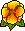## Discrete compactness for the $p$-version of discrete differential forms

Daniele Boffi, Martin Costabel, Monique Dauge, Leszek Demkowicz, Ralf HiptmairSeptember 2009. Prépublication IRMAR, 09-39

SIAM Journal on Numerical Analysis Vol. 49, No. 1, pp. 135-158 DOI: 10.1137/090772629Pdf file (340 k)    HAL    arXivIn this paper we prove the discrete compactness property for a wide class of $p$-version finite element approximations of non-elliptic variational eigenvalue problems in two and three space dimensions. In a very general framework, we find sufficient conditions for the $p$-version of a generalized discrete compactness property, which is formulated in the setting of discrete differential forms of any order on a $d$-dimensional polyhedral domain.

One of the main tools for the analysis is a recently introduced smoothed Poincaré lifting operator [M. Costabel and A. McIntosh, On Bogovskii and regularized Poincaré integral operators for de Rham complexes on Lipschitz domains, Math. Z., (2009)]. For forms of order 1 our analysis shows that several widely used families of edge finite elements satisfy the discrete compactness property in p-version and hence provide convergent solutions to the Maxwell eigenvalue problem. In particular, Nédélec elements on triangles and tetrahedra (first and second kind) and on parallelograms and parallelepipeds (first kind) are covered by our theory.